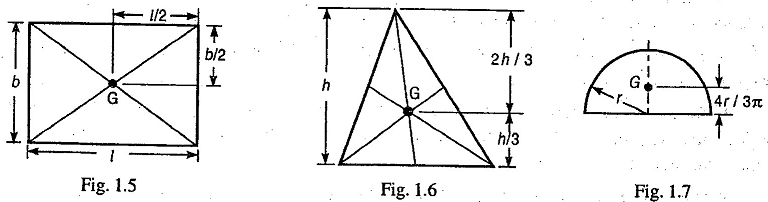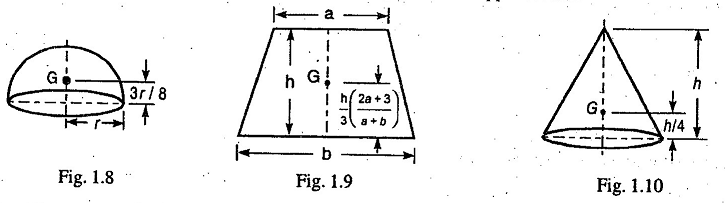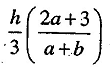# Center of Gravity

Center of Gravity (centroid) - The point through which the whole mass of the body acts, irrespective of the position of the body, is known as centre of gravity (briefly written as c.g.) The plane geometrical figures (like rectangle, triangle, circle etc.) have only areas but no mass. The centre of area of such figures is known as centroid or centre of gravity of the area of the body. It may be noted that every body has one, and only one, Centre of Gravity. The centre of gravity of some simple figures is given below.1. The center of gravity of a uniform rod is at its middle point.

2. The center of gravity (G) of a rectangle (or parallelogram) lies at a point where its diagonals intersect, as shown in Fig. 1.5.

3. The center of gravity (G) of a triangle lies at a point where the three medians of the triangle intersect, as shown in Fig. 1.6.

Note : A median is a line joining the vertex and the middle point of the opposite side.4. The center of gravity of a semi-circle lies at a distance of 4r/3π from its base measured along the vertical radius, as shown in Fig. 1.7.

5. The centre of gravity of a hemisphere lies at a distance of 3r/8 from its base, measured along the vertical radius, as shown in Fig. 1.8.

6. The centre of gravity of a trapezium with parallel sides a and b, lies at a distance ofmeasured from side b, as shown in Fig. 1.9.

7. The centre of gravity of a right circular solid cone lies at a distance of h/4 from its base, measured along the vertical axis, as shown in Fig. 1.10.

### You may like these posts:

1.1.